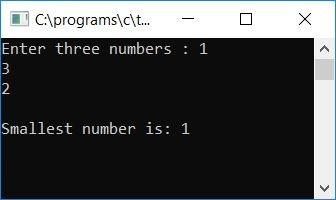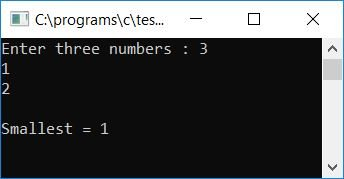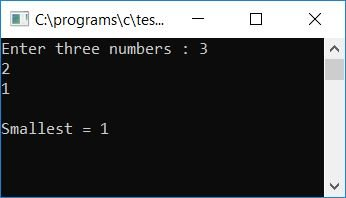# C Program to Find the Smallest of Three Numbers

Here we will learn about how to find and print the smallest among any given three numbers (by the user at run-time) with and without using any user-defined function. At the end of the article, we will also see how to find and print the smallest of three numbers using the ternary operator.

## Find the smallest of three numbers in C without a user-defined function

Here is the program that will find out the smallest of three numbers provided by the user at run-time.

```#include<stdio.h>
#include<conio.h>
int main()
{
int a, b, c, small;
printf("Enter three numbers : ");
scanf("%d%d%d", &a, &b, &c);
if(a<b)
{
if(b<c)
small = a;
else
{
if(a<c)
small = a;
else
small = c;
}
}
else
{
if(b<c)
small = b;
else
small = c;
}
printf("\nSmallest number is: %d", small);
getch();
return 0;
}```

The program was written in the Code::Blocks IDE. Here is the first snapshot of the sample run after a successful build and run:Supply any three numbers and press the ENTER key to see the smallest number among the given three numbers, as shown here in the snapshot given below:Here are some of the main steps used in the above program.

• Receive any three numbers as input, say 10, 20, and 30, inside the three variables, say a, b, and c.
• Use an if statement to determine whether a is less than b.
• If it is, then inside the if block, create another if block and check whether b is less than c.
• If it is, then initialize the value of a to any variable, say small.
• Otherwise, check whether a is less than c or not.
• If it is, then initialize a to "small."
• Otherwise, initialize c to small.
• Now come out of the if block and enter the else block (in case a is not less than b).
• Check whether b is less than c or not.
• If it is, then initialize b to small.
• Otherwise, initialize c to small.
• Now, whatever the smallest value is, small holds it.
• Therefore, just print the value of the small variable.

Here is another program to find the smallest of any three numbers in a very simple way. This program is simple and easy to understand.

```#include<stdio.h>
#include<conio.h>
int main()
{
int a, b, c, small;
printf("Enter three numbers : ");
scanf("%d%d%d", &a, &b, &c);
if(a<b && a<c)
small = a;
else if(b<a && b<c)
small = b;
else
small = c;
printf("\nSmallest number is: %d", small);
getch();
return 0;
}```

Here is the final snapshot of the above program's sample run:In the preceding program, we checked whether the first number, a, is less than both the other numbers, b and c. If it is, then we have initialized the value of a to "small". In this way, we used logic to find the smallest of three numbers in a straightforward manner.

## Using function, find the smallest of three numbers

Let's create another program that will do the same task as the above two programs have done but using user-defined functions. Here is the program that uses a user-defined function to return the smallest of any three numbers:

```#include<stdio.h>
#include<conio.h>
int getSmall(int, int, int);
int main()
{
int a, b, c, small;
printf("Enter three numbers : ");
scanf("%d%d%d", &a, &b, &c);
small = getSmall(a, b, c);
printf("\nSmallest = %d", small);
getch();
return 0;
}
int getSmall(int num1, int num2, int num3)
{
if(num1<num2)
{
if(num2<num3)
return num1;
else
{
if(num1<num3)
return num1;
else
return num3;
}
}
else
{
if(num2<num3)
return num2;
else
return num3;
}
}```

Here is the final snapshot of the sample run:## Using the ternary operator, find the smallest of three numbers

Let's create another program that will also find and print the smallest of three numbers, but this time using the ternary operator as shown in the program given below:

```#include<stdio.h>
#include<conio.h>
int main()
{
int a, b, c, small;
printf("Enter three numbers : ");
scanf("%d%d%d", &a, &b, &c);
small = (a<b) ? (a<c?a:c) : (b<c?b:c);
printf("\nSmallest = %d", small);
getch();
return 0;
}```

Here is the final snapshot of the sample run:The main logical code is:

`small = (a<b) ? (a<c?a:c) : (b<c?b:c);`

The description of the above one-line logical code is given below:

• The expression a<b returns to true.
• then the expression a<c?a:c gets executed. If this is executed and a<c returns true,
• then a is set to small and replaces the entire expression.
• Otherwise, c replaces the whole expression and will be initialized to small.
• Otherwise, the expression b<c?b:c will be executed, and if b<c returns true,
• then b replaces the whole expression and will be initialized to small.
• Otherwise, c replaces the whole expression and will be initialized to small.
• Here we have used a nested ternary operator, that is, a ternary operator inside a ternary operator, to find the smallest among three numbers.
• Assume that a, b, and c have input values of 30, 20, and 10 respectively.
• Therefore, the expression a<b or 30<20 returns to false, and then the expression b<c?b:c will be executed.
• And because b<c or 20<10 also returns to being false, c is the final value of the expression.
• Therefore, the value of c, say 10, will be initialized to small.
• Print the value of small.

C Quiz

« Previous Program Next Program »